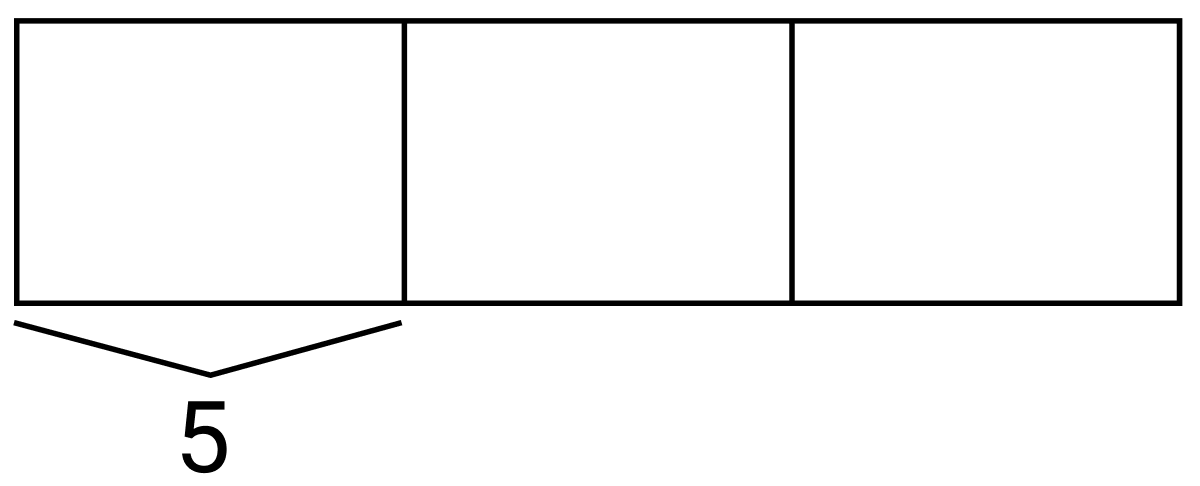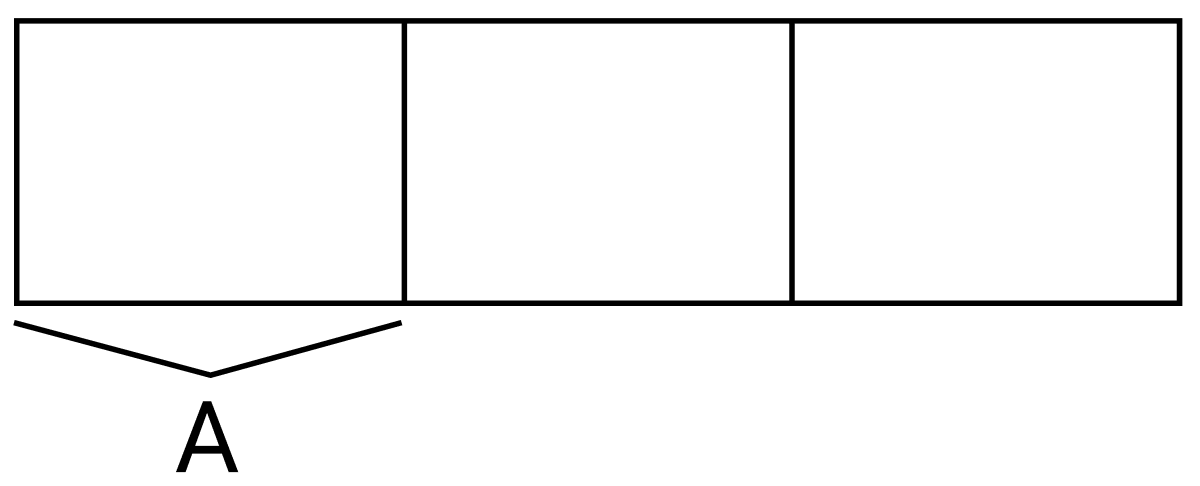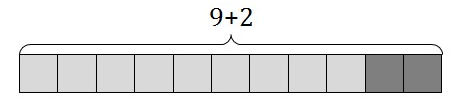# Multiplication and Division of Whole Numbers

## Objective

Write expressions that record calculations with numbers, and interpret expressions without evaluating them.

## Common Core Standards

### Core Standards

?

• 5.OA.A.1 — Use parentheses, brackets, or braces in numerical expressions, and evaluate expressions with these symbols.

• 5.OA.A.2 — Write simple expressions that record calculations with numbers, and interpret numerical expressions without evaluating them. For example, express the calculation "add 8 and 7, then multiply by 2" as 2 × (8 + 7). Recognize that 3 × (18932 + 921) is three times as large as 18932 + 921, without having to calculate the indicated sum or product.

?

• 3.OA.D.8

## Criteria for Success

?

1. Understand the sum to be the result of adding two values, the difference to be the result of subtracting two values, the product to be the result of multiplying two numbers, and the quotient to be the result of dividing two numbers.
2. Write numerical expressions based on verbal/written descriptions of calculations (e.g., write 2 x (8+7) to express the calculation “add 8 and 7, then multiply by 2”) (MP.7).
3. Write descriptions of calculations based on numerical expressions (e.g., write “add 8 and 7, then multiply by 2” to describe the expression 2 x (8+7)) (MP.7).
4. Interpret expressions without evaluating them (MP.2).

## Tips for Teachers

?

Students should be familiar with the terms sum, difference, product, and quotient from prior grade levels.

#### Fishtank Plus

• Problem Set
• Student Handout Editor
• Vocabulary Package

?

### Problem 1

Write an expression to represent the tape diagrams below. In each tape diagram, their units are equal.

1.2.#### References

EngageNY Mathematics Grade 5 Mathematics > Module 2 > Topic B > Lesson 3Concept Development

Grade 5 Mathematics > Module 2 > Topic B > Lesson 3 of the New York State Common Core Mathematics Curriculum from EngageNY and Great Minds. © 2015 Great Minds. Licensed by EngageNY of the New York State Education Department under the CC BY-NC-SA 3.0 US license. Accessed Dec. 2, 2016, 5:15 p.m..

Modified by The Match Foundation, Inc.

### Problem 2

For each problem below, write an expression that records the calculations described below, but do not evaluate.

1. 3 times the sum of 26 and 4
2. The quotient of 15 and 3 subtracted from 60

#### References

EngageNY Mathematics Grade 5 Mathematics > Module 2 > Topic B > Lesson 3Concept Development

Grade 5 Mathematics > Module 2 > Topic B > Lesson 3 of the New York State Common Core Mathematics Curriculum from EngageNY and Great Minds. © 2015 Great Minds. Licensed by EngageNY of the New York State Education Department under the CC BY-NC-SA 3.0 US license. Accessed Dec. 2, 2016, 5:15 p.m..

Modified by The Match Foundation, Inc.

### Problem 3

Write the following expressions in words:

1. $8\times(15-9)$
2. $(y+4)\div20$

#### References

EngageNY Mathematics Grade 5 Mathematics > Module 2 > Topic B > Lesson 3Concept Development

Grade 5 Mathematics > Module 2 > Topic B > Lesson 3 of the New York State Common Core Mathematics Curriculum from EngageNY and Great Minds. © 2015 Great Minds. Licensed by EngageNY of the New York State Education Department under the CC BY-NC-SA 3.0 US license. Accessed Dec. 2, 2016, 5:15 p.m..

Modified by The Match Foundation, Inc.

### Problem 4

Below is a picture that represents $9+2$.1. Draw a picture that represents $4 \times (9 + 2)$.
2. How many times bigger is the value of $4 \times (9 + 2)$ than $9+2$? Explain your reasoning.

#### References

Illustrative Mathematics Seeing is Believing

Seeing is Believing, accessed on Dec. 5, 2017, 3:52 p.m., is licensed by Illustrative Mathematics under either the CC BY 4.0 or CC BY-NC-SA 4.0. For further information, contact Illustrative Mathematics.

## Discussion of Problem Set

?

• In #3b some of you wrote 4 x (14 + 26) and others wrote (14 + 26) x 4. Are both expressions acceptable? Explain.
• Were you able to answer #4 without actually solving Expressions A and B? How?
• A student got 85 for #5a. Can you identify the error in thinking?
• Look at #6. How were you able to answer parts (b) and (c) without knowing how to compute with some of the numbers in the expressions?
• Look at #6c. Without evaluating, what would you expect the digits in the first expression to look like compared to the digits in the second expression? Why?
• We don’t yet know how to find 60 x 225. But, how were you able to use what you learned today to answer #10?

?

### Problem 1

Which phrase is represented by the expression 5 x (36 + 9)?

1. the product of 36 and 5, increased by 9
2. the product of 36 and 9, multiplied by 5
3. the sum of 36 and 9, multiplied by 5
4. the sum of 36 and 5, increased by 9

#### References

Question #7

From EngageNY.org of the New York State Education Department. New York State Testing Program Grade 5 Common Core Mathematics Test Released Questions June 2017. Internet. Available from https://www.engageny.org/resource/released-2017-3-8-ela-and-mathematics-state-test-questions/file/150271; accessed Dec. 5, 2017, 3:55 p.m..

### Problem 2

Sam divided the difference of 17 and 5 by 6. Write an expression to match Sam’s calculations.

### Problem 3

Which of the following expressions represents a number that is $3$ times larger than the sum of 8105 and 186?

a.     $({8105}+{186})\div3$

b.     $3 \times ({8105}+{186})$

c.     ${8105}+{186}\div3$

d.     $3 \times {8105}+{186}$

#### References

Massachusetts Department of Elementary and Secondary Education Spring 2013 Grade 5 Mathematics TestQuestion #14

Spring 2013 Grade 5 Mathematics Test is made available by the Massachusetts Department of Elementary and Secondary Education. © 2017 Commonwealth of Massachusetts. Accessed Dec. 5, 2017, 3:51 p.m..

?